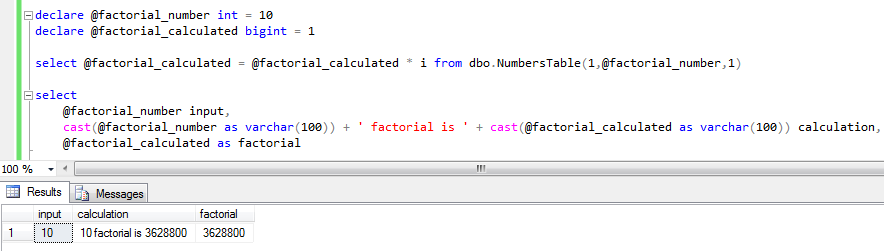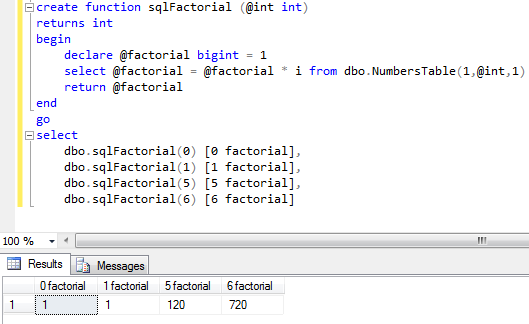SQL Server and T-SQL Development Tutorials Development resources, articles, tutorials, code samples and tools and downloads for ASP.Net, SQL Server, R Script, Windows, Windows Phone, AWS, SAP HANA and ABAP, like SAP UI5, Screen Personas, etc.
 Home Articles News IT Jobs Tools Sample Chapters Trainers Blogs Forums Photos Files

SQL Server 2019 Installation

# SQL Factorial Function to Calculate Factorial of an Integer in SQL Server

In SQL Server, developers can use SQL factorial function given in this tutorial to calculate factorial for a given integer value. For mathematical factorial calculation of an integer input value in SQL Server, sqlFactorial() user-defined function can be used as shown in the SQL tutorial.

If you don't already have a numbers table in your database, you can use following SQL codes to calculate factorial value of an input integer value.

``` -- let's calculate 6 factorial declare @factorial_number int = 6 declare @factorial_calculated bigint = 1 select top (@factorial_number)  @factorial_calculated = @factorial_calculated * ROW_NUMBER() over (order by id) from sys.sysobjects order by id select @factorial_calculated -- result, factorial of @factorial_number ```

Let's see the resultsOn the other hand, if you have a numbers table in your SQL Server database the above code can be converted into a more readable SQL script as follows:

``` -- let's calculate 10 factorial declare @factorial_number int = 6 declare @factorial_calculated bigint = 1 select @factorial_calculated = @factorial_calculated * i from dbo.NumbersTable(1,@factorial_number,1) select @factorial_calculated -- (@factorial_number) factorial ```

SQL programmers can check SQL tutorial Create Numbers Table in SQL Server for dbo.NumbersTable() table valued function codes

Here is the output for 10! calculation (10 factorial calculation) in SQL Server for programmers.Of course it is easier to convert the second method into a SQL factorial function to calculate factorial values easily.

``` create function sqlFactorial (@int int) returns int begin  declare @factorial bigint = 1  select @factorial = @factorial * i from dbo.NumbersTable(1,@int,1)  return @factorial end go select  dbo.sqlFactorial(0) [0 factorial],  dbo.sqlFactorial(1) [1 factorial],  dbo.sqlFactorial(5) [5 factorial],  dbo.sqlFactorial(6) [6 factorial] ```

Here is how SQL programmers can use sqlFactorial user-defined function (UDF) to calculate factorial value of an input positive integer value.Related SQL Resources

SQL Server Articles

SQL Server 2012

SQL Server Tools

SQL Blog

MS SQL Server Forums